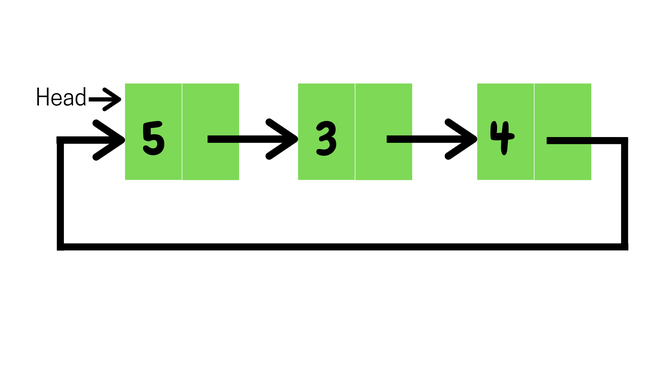Open in App
Not now

# Java Program to Delete a Node From the Ending of the Circular Linked List

• Last Updated : 21 Aug, 2022Example:

```Input : 5->3->4->(head node)

We will first initialize the list and add some data into it with the addNode() method and then proceed according to the below approaches:

Case 1: List is empty.

If the list is empty we will simply return.

Case 2: List is not empty

• delete():
• Return from the function if no node is present
• Sets both head and tail to null if only one node is there
• if it has more than one node then it removes the previous head node, the head will point to the next node in the list and tail will point to the new head.
• printNode() will print all the nodes present in the list as:
• Node current is defined which will point to the head
• Print current.val till it starts pointing to the head again
• In each iteration, it will point to the next node

Code:

## Java

 `// Java Program to Delete a Node From the Ending of the``// Circular Linked List` `public` `class` `Main {``    ``// Represents the node of list.``    ``public` `class` `Node {``        ``int` `val;``        ``Node next;``        ``public` `Node(``int` `val) { ``this``.val = val; }``    ``}` `    ``// Initialising head and tail pointers``    ``public` `Node head = ``null``;``    ``public` `Node tail = ``null``;` `    ``// add new node to the end``    ``public` `void` `addNode(``int` `val)``    ``{``        ``// Creating new node``        ``Node node = ``new` `Node(val);` `        ``// head and tail will point to new node``        ``// if list is empty``        ``if` `(head == ``null``) {``            ``head = node;``            ``tail = node;``            ``node.next = head;``        ``}``        ``// otherwise tail point to new node and``        ``else` `{``            ``tail.next = node;``            ``tail = node;``            ``tail.next = head;``        ``}``    ``}` `    ``// Deletes node from the beginning of the list``    ``public` `void` `delete()``    ``{``        ``// returns if list is empty``        ``if` `(head == ``null``) {``            ``return``;``        ``}``        ``// otherwise head will point to next element in the``        ``// list and tail will point to new head``        ``else` `{``            ``// if list contains more than one element``            ``// then loop will iterate``            ``// till second last element``            ``// is pointing to tail``            ``if` `(head != tail) {``                ``Node current = head;``                ``while` `(current.next != tail) {``                    ``current = current.next;``                ``}``                ``// Second last element is the new tail``                ``tail = current;``                ``tail.next = head;``            ``}` `            ``// if the list contains only one element``            ``// then both head and tail will point to null``            ``else` `{``                ``head = tail = ``null``;``            ``}``        ``}``    ``}` `    ``// displaying the nodes``    ``public` `void` `printNode()``    ``{``        ``Node current = head;``        ``if` `(head == ``null``) {``            ``System.out.println(``"List is empty"``);``        ``}``        ``else` `{``            ``do` `{``                ``System.out.print(``" "` `+ current.val);``                ``current = current.next;``            ``} ``while` `(current != head);``            ``System.out.println();``        ``}``    ``}` `    ``public` `static` `void` `main(String[] args)``    ``{``        ``Main list = ``new` `Main();` `        ``// Adds data to the list``        ``list.addNode(``5``);``        ``list.addNode(``3``);``        ``list.addNode(``4``);` `        ``// Printing original list``        ``System.out.println(``"Original List: "``);``        ``list.printNode();` `        ``// deleting node from ending``        ``// till the list is empty and``        ``// displaying the Updated list``        ``while` `(list.head != ``null``) {``          ` `            ``list.delete();``          ` `            ``// Printing updated list``            ``System.out.println(``"Updated List: "``);``            ``list.printNode();``        ``}``    ``}``}`

Output

```Original List:
5 3 4
Updated List:
5 3
Updated List:
5
Updated List:
List is empty```

Time complexity: O(n) where n is no of nodes of circular linked list

Auxiliary Space: O(1)

My Personal Notes arrow_drop_up